Courses
Courses for Kids
Free study material
Free LIVE classes
More

# Liquid Measurement - Different Units to Measure LiquidsLIVE
Join Vedantu’s FREE Mastercalss

## What is Liquid Measurement?

Liquid measurement is the amount of liquid a vessel or container holds and its measurement in standard units. This is also referred to as capacity or the volume of the vessel. The two most common units of measuring liquid are:

• Millilitres

• Litres

## What is Millilitre?

A millilitre is the smallest metric unit that measures the smallest quantity of liquid and is equal to a thousandth of a litre. Hence, we can say 1 litre = 1000 millilitres. The figure below shows the 1000 millilitres of water in a jug.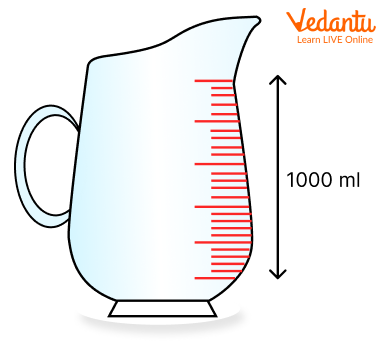Jug with 1000 ml of water

• A single raindrop contains about 1 millilitre of liquid.

• 20 drops of water make about 1 millilitre of liquid.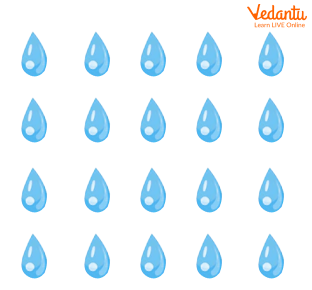20 drops of water makes about 1 millilitre of liquid

• One teaspoon of liquid makes about 5 millilitres.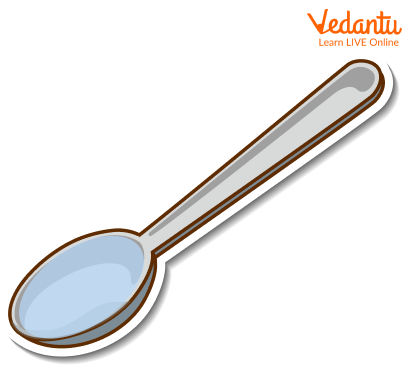One teaspoon of liquid makes about 5 millilitres

## How is Millilitre Written in Short?

In short, millilitres are often written as Ml or ML. Hence, 100 Ml or ML is written as 100 millilitres.

Note:

One millilitre is also equivalent to 1 cubic centimetre.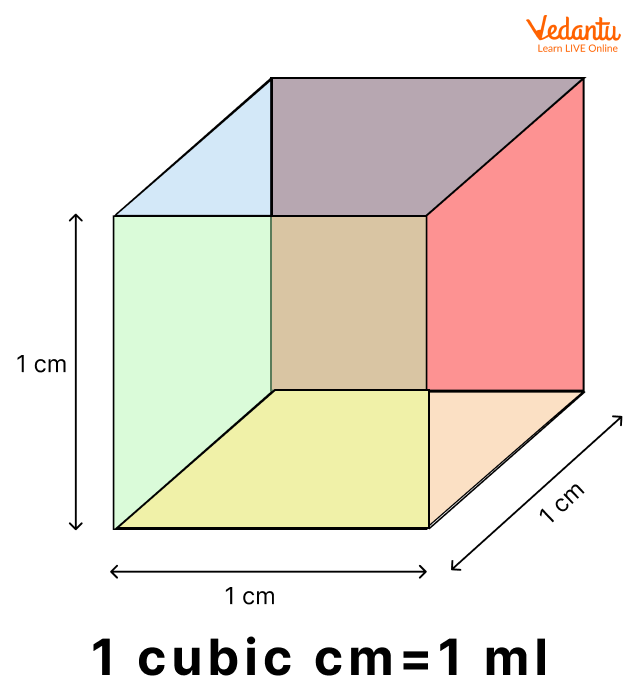1ml = 1 cubic cm

In other words, we can say 1 millilitre is the same as a little cube that is 1 cm on each side (1 cubic centimetre).

## What is Litre?

A litre is another basic metric unit that is used to measure the capacity of liquid. The figure below shows the 1 litre of water in a jug.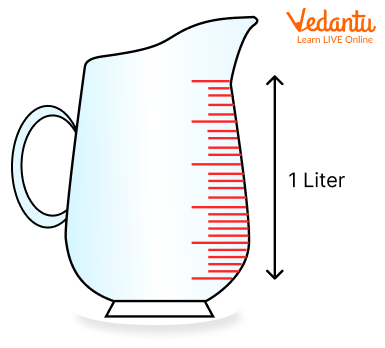Jug with 1 litre of water

## How is Litre Written in Short?

In short, litre is often written as L or l. Hence, 3 L or 3l means 3 litres.

Milk, soda, juices, and other drinks are often sold in litres.

Note:

• 1 litre is equal to one cubic decimetre, or 1 L = 1 dm³.

• 1 cube is equivalent to 0.1 metres or 10 cm or 10 mm on each side containing 1 litre.

• A square metre i.e. 1 millilitre thick also contains 1 litre.

## Other Liquid Measurements

Cubic Millilitre

A cube with one millimetre on each side is equivalent to 1 cubic millilitre. Hence, we need 1000 cubic millimetres to make 1 cubic millilitre. 1 cubic millilitre is also equal to one-millionth of a litre or one-billionth of a cubic metre.

Cubic Centimetre

1 cubic centimetre, written as cm³, which is a cube that is 1 cm on each side. It is also equivalent to 1 ML. Hence, 1 cm³ = 1 ML.

1 cubic centimetre is also one-thousandth of a litre or one-millionth of a cubic metre.

Decilitre

A decilitre, written as dl, is 1/10 of a litre or 100 ml. Hence, 1 dl = 100 ml.

Cubic Metre

1 Cubic metre, written as m³, is a cube with 1 metre on each side. Also, 1 m³ = 1000 litres.

Megalitre

1 Megalitre, written as ML, is useful for measuring large quantities of water, such as in dams or in small lakes. 1 megalitre is one million litres or 1 megalitres = 1,000,000 litres. Also, 1 megalitre (ML) = 1000 m³.

Cubic Kilometre

A Cubic Kilometre is useful for measuring large lakes, seas, and oceans. It is written as Km³, is a cube that is 1 Kilometre on each side. 1 Cubic Kilometre is equivalent to 1 billion m³. It can also be written as 1 Km³ = 1,000,000,000 cubic metres.

## Liquid Measurement Tools

The different tools for measuring liquid are:

• Measuring cups and pitchers

• Laboratory essentials

Measuring Cups and Pitchers: We generally use cups and pitchers with markings in the kitchen to measure the quantity of liquid. The pictures given below show different cups and pitchers in the kitchen.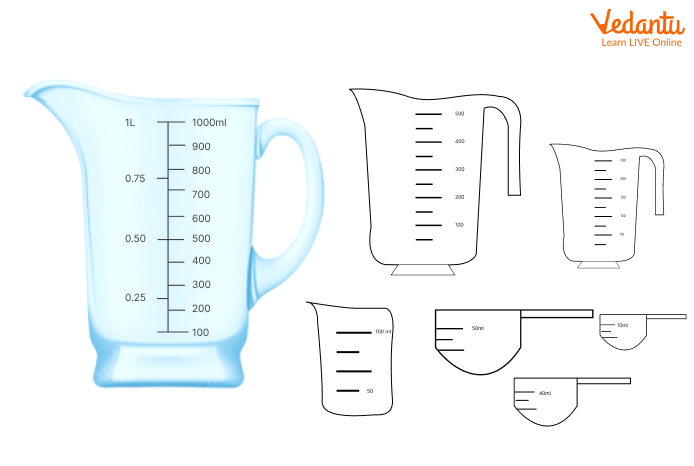Measuring cups

Laboratory Essentials: The liquid measurement equipment such as beakers, conical flask, test tubes, and graduated cylinders with permanent markings in metric or customary are used to measure liquids precisely for performing tests with chemicals and other liquid compounds. The picture given below shows a few laboratory essentials with markings.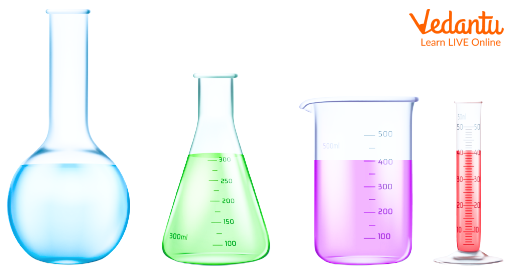Laboratory Essentials

## Common Metric and US Metric Conversion for Measuring Liquids

The table below shows some common metric and US metric conversions for measuring the volume of liquids.

 Unit Equivalent 1 Fluid Ounce 29.57 millilitres 1 Cup 236.588 millilitres 1 Quart 946.353 millilitres 1 Millilitre 0.034 Fluid Ounces 1 litre 33.8 Fluid Ounces

## Did You Know?

• The Pacific ocean holds almost 700,000,000 Km³ of water.

• Lake Bikanel, widely known as the largest continental lake in the world, holds almost 23,600 Km³ of water.

• 1 tablespoon is equivalent to 3 teaspoons of 15 millilitres.

• 1 litre is very close to the volume of a kilogram of water.

• A millilitre is about the size of a grape.

## Conclusion

In short, liquid measurement is defined as the amount of liquid a vessel holds and its measurement in standard units. In other words, it is also referred to as the volume or amount of vessel. Infant milk bottle with a millimetre marking is an example of liquid measurement.

Last updated date: 17th Sep 2023
Total views: 130.2k
Views today: 1.30k

## FAQs on Liquid Measurement - Different Units to Measure Liquids

1. How to measure the volume of liquid?

To measure the volume of liquid, you can use measuring cups or spoons. Liquid measuring cups are generally made up of plastics or glass, and most of them have spouts for pouring. Ensure not to use a dry measuring cup for measuring liquid as it may not give you an accurate measurement.

2. What are the most common units used for measuring liquids?

The two most common units for measuring liquids are litres and millilitres. A litre is the standard unit of measuring liquid in a metric system.

3. How do we measure liquid ingredients precisely?

To measure liquid ingredients precisely, ensure to use a liquid measuring with a pouring spout. Also ensure to choose clear measuring cups with large, easy-to-read markings. Place the measuring cup flat on the countertop or table. Also, use a rubber spatula or spoon.